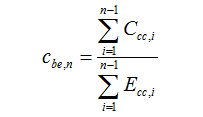# HOMER Grid 1.3Type: Intermediate Variable Units: \$/kWh Symbol: cbe,n

In any time step, the storage energy cost is the average cost of the energy that the system has put into the storage bank up until that time step. HOMER uses the following equation to calculate the storage energy cost in each time step:[\$]

 where: cbe,n = the storage energy cost in time step n [\$/kWh] Ccc,i = the cost of cycle charging the storage in time step i Ebc,i = the amount of energy that went into the storage bank in time step i [kWh]

The storage energy cost reflects the average cost that the system has incurred for deliberately charging the storage bank. The "cost of cycle charging," which appears in the numerator of the above equation, is the extra cost incurred by the system specifically for charging the storage. Excess electricity that charges the storage bank in some time step represents no such cost. But if the generator produced more power than required to serve the load, and it did so specifically to charge the storage, then that act of charging the storage bank does cause the system to incur extra cost. The same is true if the system purchases extra grid power expressly to charge the storage. Such events occur routinely under the cycle charging strategy.

In any time step in which a generator or the grid cycle charges the storage, HOMER calculates the cycle charge cost by taking the actual cost of operating the system in that time step and subtracting the cost that would have occurred in that time step had the system not charged the storage.

Under the load following dispatch strategy, the storage energy cost is always zero because the system never pays to charge the storage bank; it only uses excess electricity to charge the storage bank.

Tip: The storage bank's marginal cost of generation is equal to the sum of the storage wear cost and the storage energy cost.